Home

# Phoretic self-propulsion at large Péclet numbers

## Abstract

We analyse the self-diffusiophoresis of a spherical particle animated by a non-uniform chemical reaction at its boundary. We consider two models of solute absorption, one with a specified distribution of interfacial solute flux and one where this flux is governed by first-order kinetics with a specified distribution of rate constant. We employ a macroscale model where the short-range interaction of the solute with the particle boundary is represented by an effective slip condition. The solute transport is governed by an advection–diffusion equation. We focus upon the singular limit of large Péclet numbers,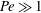$\mathit{Pe}\gg 1$ . In the fixed-flux model, the excess-solute concentration is confined to a narrow boundary layer. The scaling pertinent to that limit allows the problem governing the solute concentration to be decoupled from the flow field. The resulting nonlinear boundary-layer problem is handled using a transformation to stream-function coordinates and a subsequent application of Fourier transforms, and is thereby reduced to a nonlinear integral equation governing the interfacial concentration. Its solution provides the requisite approximation for the particle velocity, which scales as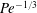$\mathit{Pe}^{-1/3}$ . In the fixed-rate model, large Péclet numbers may be realized in different limit processes. We consider the case of large swimmers or strong reaction, where the Damköhler number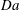$\mathit{Da}$ is large as well, scaling as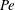$\mathit{Pe}$ . In that double limit, where no boundary layer is formed, we obtain a closed-form approximation for the particle velocity, expressed as a nonlinear functional of the rate-constant distribution; this velocity scales as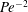$\mathit{Pe}^{-2}$ . Both the fixed-flux and fixed-rate asymptotic predictions agree with the numerical values provided by computational solutions of the nonlinear transport problem.

## References

Hide All
MathJax
MathJax is a JavaScript display engine for mathematics. For more information see http://www.mathjax.org.

# Phoretic self-propulsion at large Péclet numbers

## Metrics

### Full text viewsFull text views reflects the number of PDF downloads, PDFs sent to Google Drive, Dropbox and Kindle and HTML full text views.

Total number of HTML views: 0
Total number of PDF views: 0 *Loading metrics...

### Abstract viewsAbstract views reflect the number of visits to the article landing page.

Total abstract views: 0 *Loading metrics...

* Views captured on Cambridge Core between <date>. This data will be updated every 24 hours.

Usage data cannot currently be displayed.